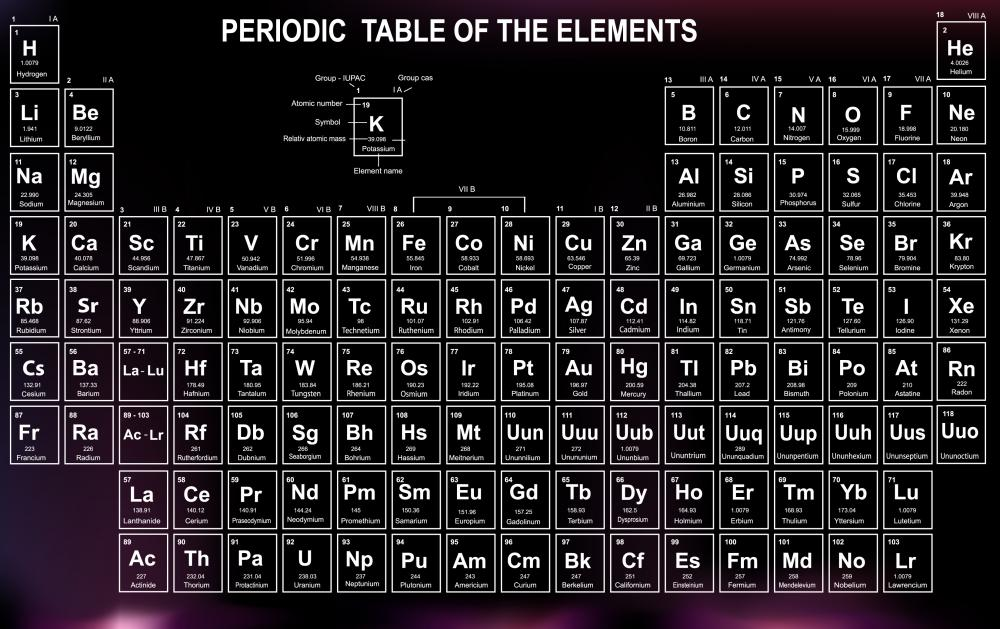# What are Nuclides?

Angie Bates

Nuclides are atoms with a set number of protons and neutrons. Usually an isotope of an element, they can be stable or unstable. Unstable nuclides are radioactive. There are about 1,700 known nuclides, 1,400 of which are radioactive.Each element on the Period Table is a nuclide, as are all the isotopes of each element.

The terms "nuclide" and "isotope" are sometimes used interchangeably, but they are not synonymous. Isotopes are sets of the same element that have differing numbers of neutrons. Isotopes are nuclides, but nuclides are not necessarily isotopes.

Elements are defined by the number of protons they possess. The Periodic Table of Elements is arranged by the number of protons in each element. Each element on the Period Table is a nuclide, as are all the isotopes of each element.

The scientific notion for a nuclide is usually written AZX. The X stands for the element's symbol, the Z for the number of protons, or the atomic number, and the A stands for the mass number, or the number of protons plus neutrons in the element. For example, lithium's notion is written 73Li. This tells us that lithium contains three protons and four neutrons, since 7 minus 3 is 4. Lithium also has several isotopes, such as 63L, written as lithium-6. Lithium-6 contains only three neutrons.

Protons naturally repel each other because they all carry a positive charge, so the stabilizing influence of the neutrons is what keeps an element intact. If the nuclide is light, it is usually stable when the number of protons and neutrons are equal or near equal. The heavier the element, the greater the ratio of neutrons to protons needed to make it stable.

Unstable nuclides are radioactive and are either naturally occurring or artificially made. There are three types of radiation that an unstable nuclide emits: alpha or beta particles or gamma rays. Alpha and beta particles are fairly harmless because they can be easily prevented from entering the body. Gamma rays, however, are extremely harmful and are why radioactive elements are considered so dangerous. The breakdown of an unstable nuclide is called radioactive decay.

An unstable nuclide eventually decays into a stable nuclide. Each radioactive nuclide has a fixed rate of decay, known as its half-life. Knowing the half—life of certain elements and measuring ratios of stable versus unstable nuclides present in a given object allows scientists to date fossils and artifacts.

Nuclear power plants also use nuclides to create energy by a process called fission. Occurring in a nuclear reactor, fission breaks apart heavier nuclides into lighter ones. This reaction releases energy which is harnessed by the plant. Nuclear power is often considered dangerous because the nuclide used in the fission process, Uranium-235, is a radioactive isotope.

## You might also Like